# Automatic garden irrigator (AGI)

By analyzing atmospheric values, this tiny unit can decide when to irrigate your garden if it is necessary.

IntermediateWork in progress20 hours1,790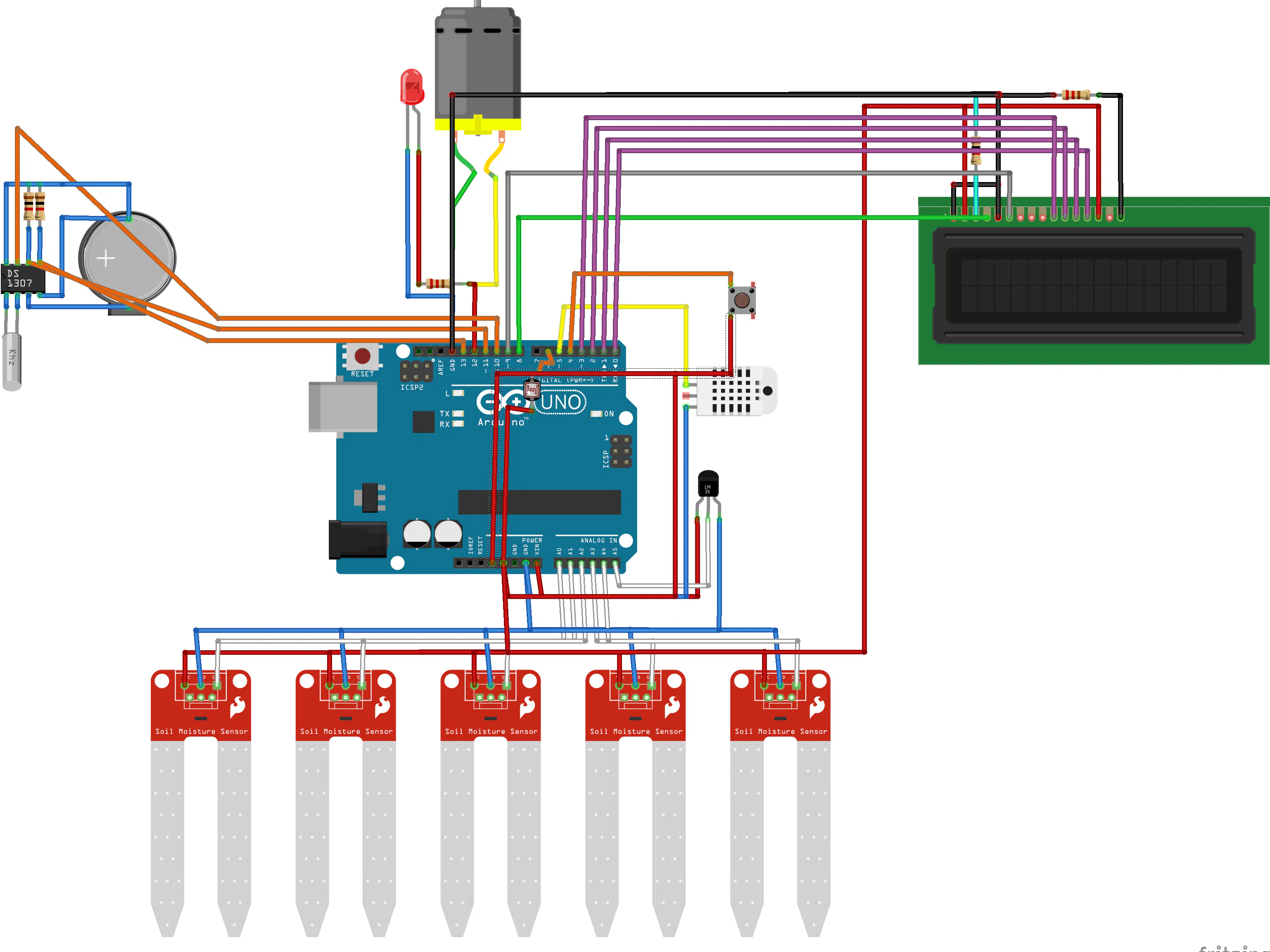## Things used in this project

### Hardware componentsArduino UNO & Genuino UNO
×1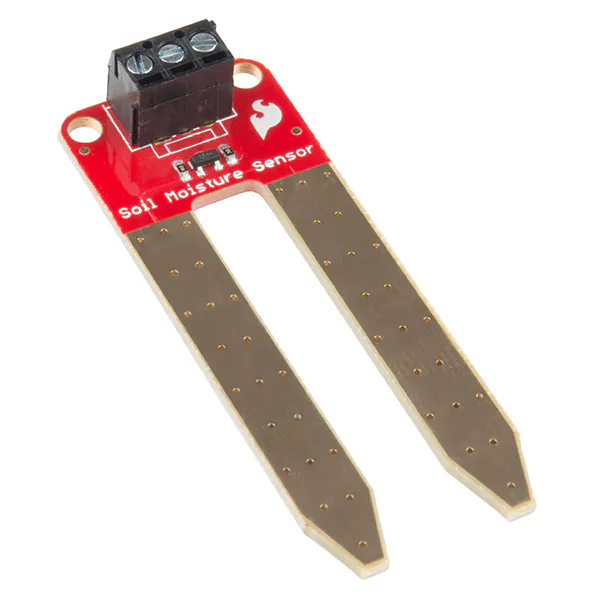SparkFun Soil Moisture Sensor (with Screw Terminals)
×8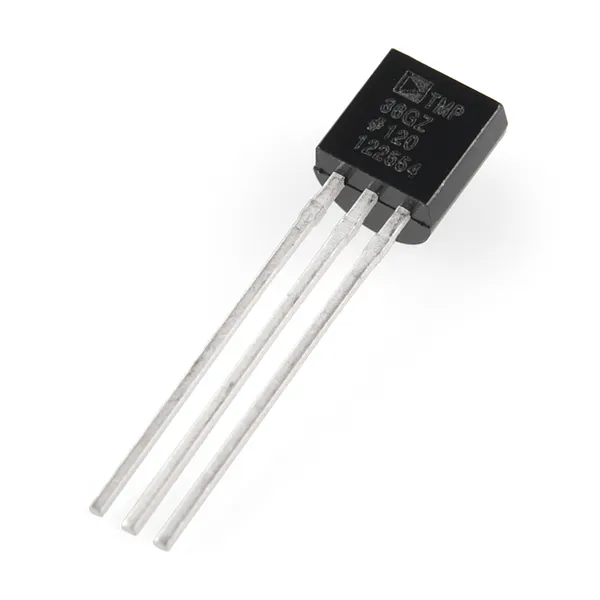Temperature Sensor
×1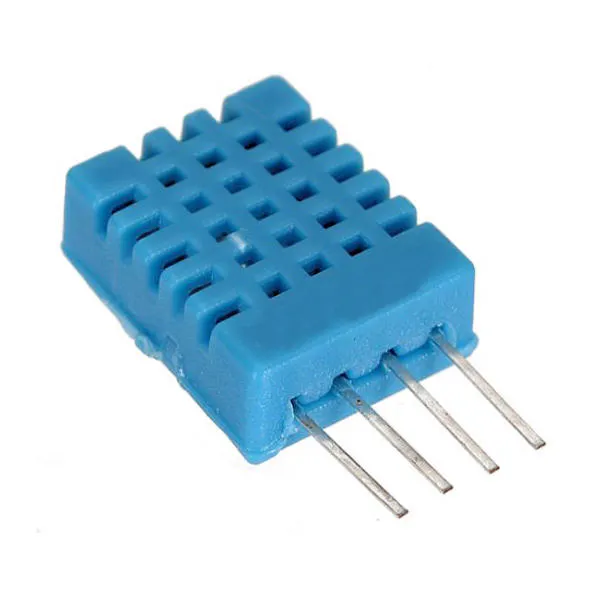DHT11 Temperature & Humidity Sensor (4 pins)
×1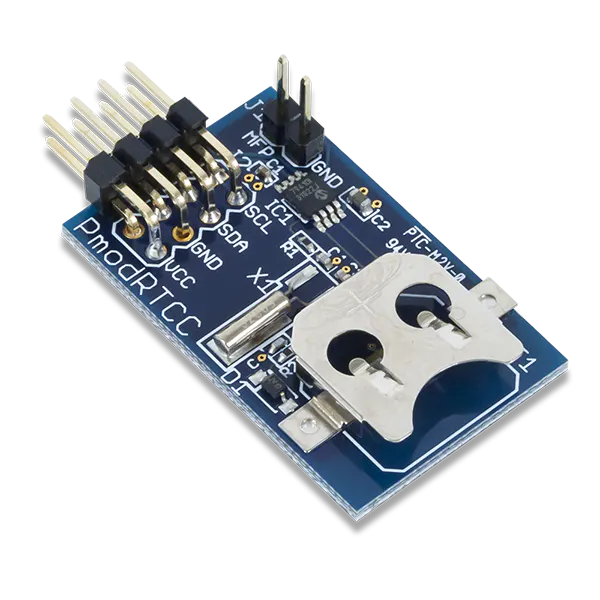Digilent Pmod RTCC
×1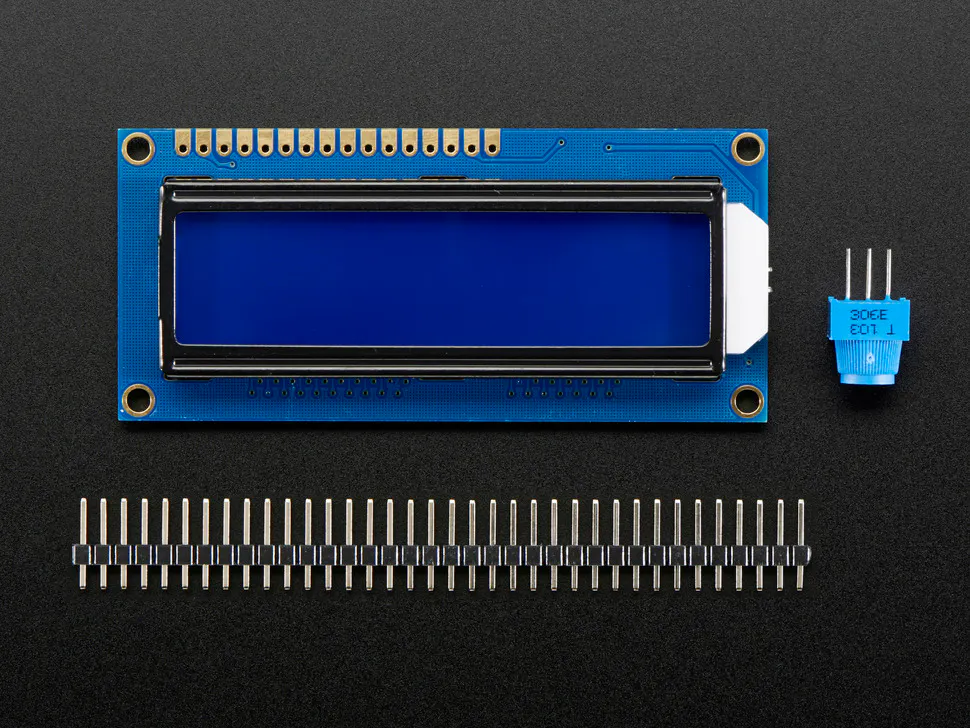Adafruit Standard LCD - 16x2 White on Blue
×1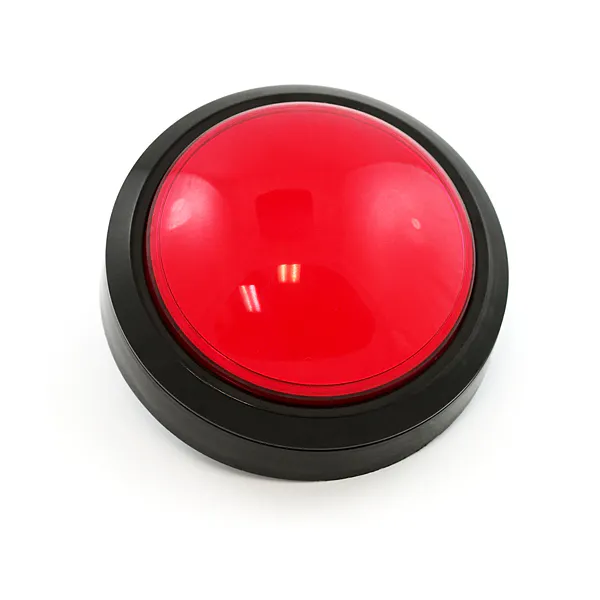SparkFun Big Red Dome Button
×1LED (generic)
×1

### Software apps and online servicesArduino IDE
 fritzing

 welder

## Schematics

### schematics of AGI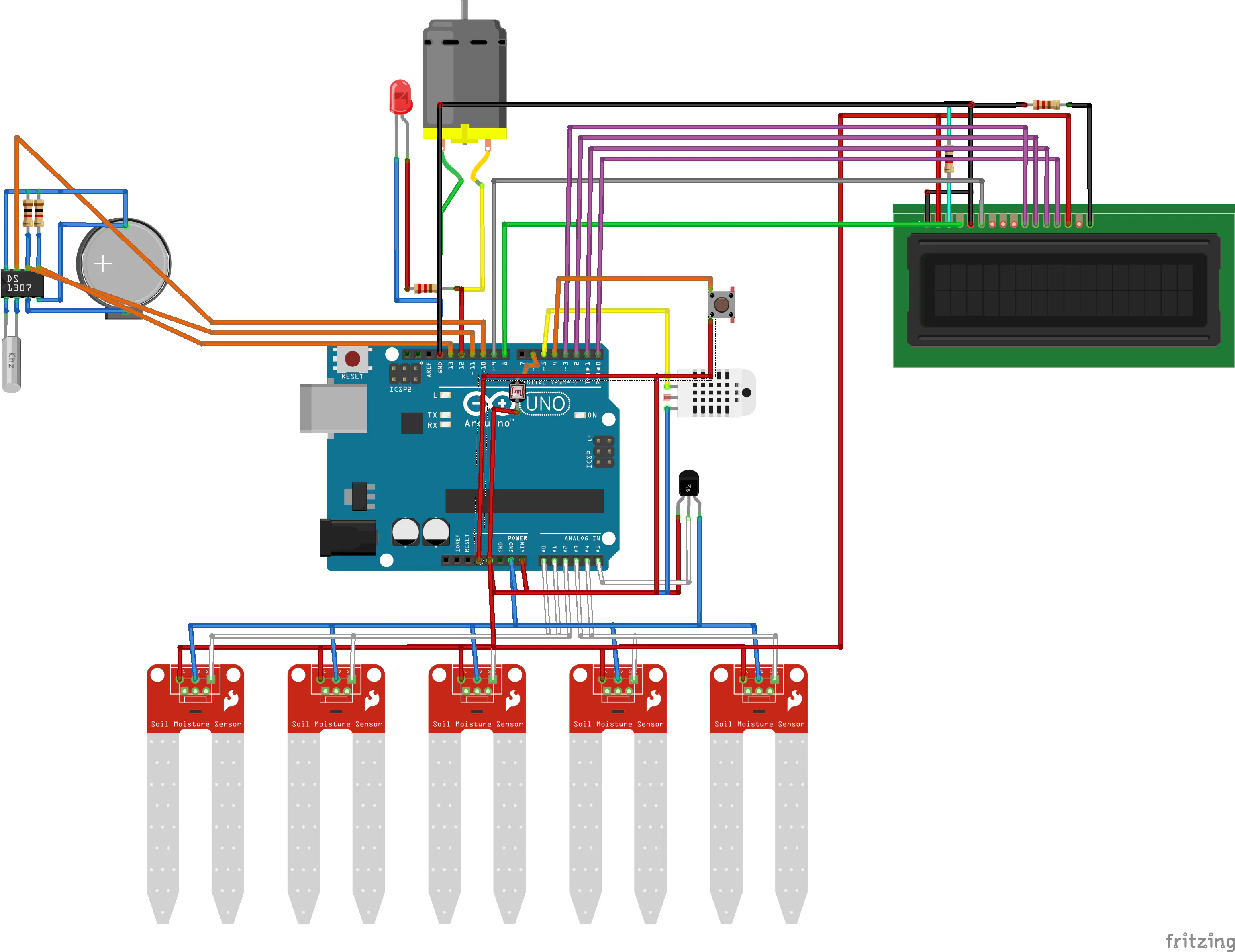## Code

### AGI code

C/C++
```#include <LiquidCrystal.h>
#include <Wire.h>
#include "RTClib.h"

#define TEMP A5 //LM35
#define H1 A0 //igrometro 1
#define H2 A1 //igrometro 2
#define H3 A2 //igrometro 3
#define H4 A3 //igrometro 4
#define H5 A4 //igrometro 5
#define HUM 5 //umidità
#define LHT 6 //intensità luminosa
#define BTN 4 //controllo display
#define MOTOR 12 //pompa acqua
#define SDA 10 //sda rtc
#define SCL 11 //scl rtc

int x = 0;
bool innaffiato = false;
LiquidCrystal lcd (8, 9, 3, 2, 1, 0);
RTC_DS1307 rtc;

void setup() {
lcd.begin(16, 2);
Serial.begin(9600);
rtc.begin();
Wire.begin();

lcd.setCursor(0, 0);
lcd.print("initialization");
lcd.setCursor(0, 1);

pinMode(TEMP, INPUT);
pinMode(H1, INPUT);
pinMode(H2, INPUT);
pinMode(H3, INPUT);
pinMode(H4, INPUT);
pinMode(H5, INPUT);
pinMode(HUM, INPUT);
pinMode(LHT, INPUT);
pinMode(BTN, INPUT);
pinMode(MOTOR, OUTPUT);
if (! rtc.isrunning()) {
Serial.println("RTC is NOT running!");
// following line sets the RTC to the date & time this sketch was compiled
}
else
{
//    rtc.DateTime(2018, 04, 18, 11, 55, 0);
}

delay(1000);
lcd.clear();
}

void loop() {
DateTime _now = rtc.now();
float t = analogRead(TEMP) / 2.046;
float hyg1 = map(analogRead(H1), 1023, 350, 0, 100);
float hyg2 = map(analogRead(H2), 1023, 350, 0, 100);
float hyg3 = map(analogRead(H3), 1023, 350, 0, 100);
float hyg4 = map(analogRead(H4), 1023, 350, 0, 100);
float hyg5 = map(analogRead(H5), 1023, 350, 0, 100);
float hm = map(analogRead(HUM), 1023, 350, 0, 100);
float light = map(analogRead(LHT), 1023, 350, 0, 100);

x++;
if (x >= 9)
x = 0;
switch (x)
{
case 1:
lcd.clear();
lcd.setCursor(0, 0);
lcd.print("Temperatura");
lcd.setCursor(0, 1);
lcd.print(t + '%'); //1 temperatura
break;
case 2:
lcd.clear();
lcd.setCursor(0, 0);
lcd.print("igrometro 1");
lcd.setCursor(0, 1);
lcd.print(hyg1 + '%');
break;
case 3:
lcd.clear();
lcd.setCursor(0, 0);
lcd.print("igrometro 2");
lcd.setCursor(0, 1);
lcd.print(hyg2 + '%');
break;
case 4:
lcd.clear();
lcd.setCursor(0, 0);
lcd.print("igrometro 3");
lcd.setCursor(0, 1);
lcd.print(hyg3 + '%');
break;
case 5:
lcd.clear();
lcd.setCursor(0, 0);
lcd.print("igrometro 4");
lcd.setCursor(0, 1);
lcd.print(hyg4 + '%');
break;
case 6:
lcd.clear();
lcd.setCursor(0, 0);
lcd.print("igrometro 5");
lcd.setCursor(0, 1);
lcd.print(hyg5 + '%');
break;
case 7:
lcd.clear();
lcd.setCursor(0, 0);
lcd.print("Umidita");
lcd.setCursor(0, 1);
lcd.print(hm + '%');
break;
case 8:
lcd.clear();
lcd.setCursor(0, 0);
lcd.print("Luminosita");
lcd.setCursor(0, 1);
lcd.print(light + '%');
break;
}
if (((light < 25) && (innaffiato == false)) && ((hyg1 < 30) || (hyg2 < 30) || (hyg3 < 30) || (hyg4 < 30) || (hyg5 < 30)) && ((_now.hour() > 17) && (_now.hour() < 23)))
digitalWrite(MOTOR, HIGH);

if ((hyg1 > 80) && (hyg2 > 80) && (hyg3 > 80) && (hyg4 > 80) && (hyg5 > 80))
{
digitalWrite(MOTOR, LOW);
innaffiato = true;
}
if (_now.hour() == 1)
innaffiato = false;
}
```

## Credits

### Daniel Rossi

1 project • 12 followers
i am a student of IT engineering at the university of modena and reggio emilia. Instagram: @danielrossitv- @officialprojecto